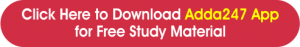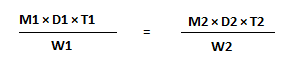Home   »   Time and Work Questions, Formulas, Examples

# Time and Work Questions, Formulas, Examples

## Time and Work

In any competitive exam where there is a section of quantitative aptitude, there is a high possibility of having Time and Work questions included in it. You will find such questions generally in Government exams. And before facing any competitive exam, everyone once go through such questions in their school time and it’s considered one of the easiest and interesting topic of Math.

In every type of time and work questions, concept remains the same, but the language changes as per the different type of framing of these questions. At least, 2-3 questions are asked in any competitive exam. If they are solved with proper trick, they can be solved in no time.

Mostly aspirants are fearful due to the difficulty level of math and many struggle a lot to actually able to get required marks in this section. But as we know in this case using proper tricks and lots of practice is the key to gain marks because no matter how tough it can be, reality is, it is the most scoring subject too among all the subjects as there is certainty about ithe answers, unlike, others subjects where there is a high chance of losing marks due to uncertainty. So With the clear and basic understanding of the topic and with the proper practice, one can easily ace the exam.

In this article we will try to understand the concept of Time and Work in order to clear the basics and score maximum in this section. Also, we will add few sample time and work questions with solutions for your reference.

## Concept of Time and Work

As we have discussed that understanding the concept is the m=foremost thing for the competitive exams. So talking about its components, as clear by name, there are two – Time and Work. Basically it  means how much time has been taken to complete a given amount of work. It can be completed by any individual or a group of individual, generally the number of people completing the work is also given and it’s also considered as one of the component.## Types of Questions in Time and Work

Here we will discuss the basic type of questions which may be asked in the exam with respect to the time and work topic:

• Finding out the amount of work done in a specified time by an individual or a group
• Finding out the time taken to complete the work by an individual or a group
• Efficiency of the particular persons involved in the task

Whenever you will come across such questions, it will be based on the above mentioned concept. Nothing out of this is possible to be asked.

## Time and Work Formulas

As we know it’s important to be aware of the formula to be used for some specific questions. A candidate needs to be well versed with the formulas so that one can find the solution in the least time possible. Here, we will talk about the formulas to be used solve questions related to Time and Work.

• Total Work Done = Time Taken × Rate of Work
• Rate of Work = 1 / Time Taken
• Time Taken = 1 / Rate of Work
• If a piece of work is done in x number of days, then the work done in one day = 1/x
• Total Wok Done = Number of Days × Efficiency

## Time and Work: Important Points to Be Noticed

1. Efficiency and Time are inversely proportional to each other.
2. X:y is the ratio of the number of men which are required to complete a piece of work, then the ratio of the time taken by them to complete the work will be y:x
3. If x number of people can do W1 work, in D1 days, working T1 hours each day and the number of people can do W2 work, in D2 days, working T2 hours each day, then the relation between them will beAnalyse the information given in the statement form in the question, put the given data in the formula and find out what has been asked.

## Time and Work Questions

A takes twice as much time as B or thrice as much time as C to finish a piece of work. Working together, they can finish the work in 2 days. B can do the work alone in:
 A. 4 days B. 6 days C. 8 days D. 12 days

A is 30% more efficient than B. How much time will they, working together, take to complete a job which A alone could have done in 23 days?
A. 11 days
B. 13 days
C.
 20 3 days 17
D. None of these
4 men and 6 women can complete a work in 8 days, while 3 men and 7 women can complete it in 10 days. In how many days will 10 women complete it?
 A. 35 B. 40 C. 45 D. 50
A can do a certain work in the same time in which B and C together can do it. If A and B together could do it in 10 days and C alone in 50 days, then B alone could do it in:
 A. 15 days B. 20 days C. 25 days D. 30 days
A, B and C can do a piece of work in 20, 30 and 60 days respectively. In how many days can A do the work if he is assisted by B and C on every third day?
 A. 12 days B. 15 days C. 16 days D. 18 daysThank You, Your details have been submitted we will get back to you.
•AFCAT 2 Exam Analysis 2022 : 28th August...
•AFCAT 2 Exam Analysis 2022 : 28th August...
•AFCAT Exam Analysis 2022, 27th August Sh...